# Experiment to make the LED a "glowing light sensor"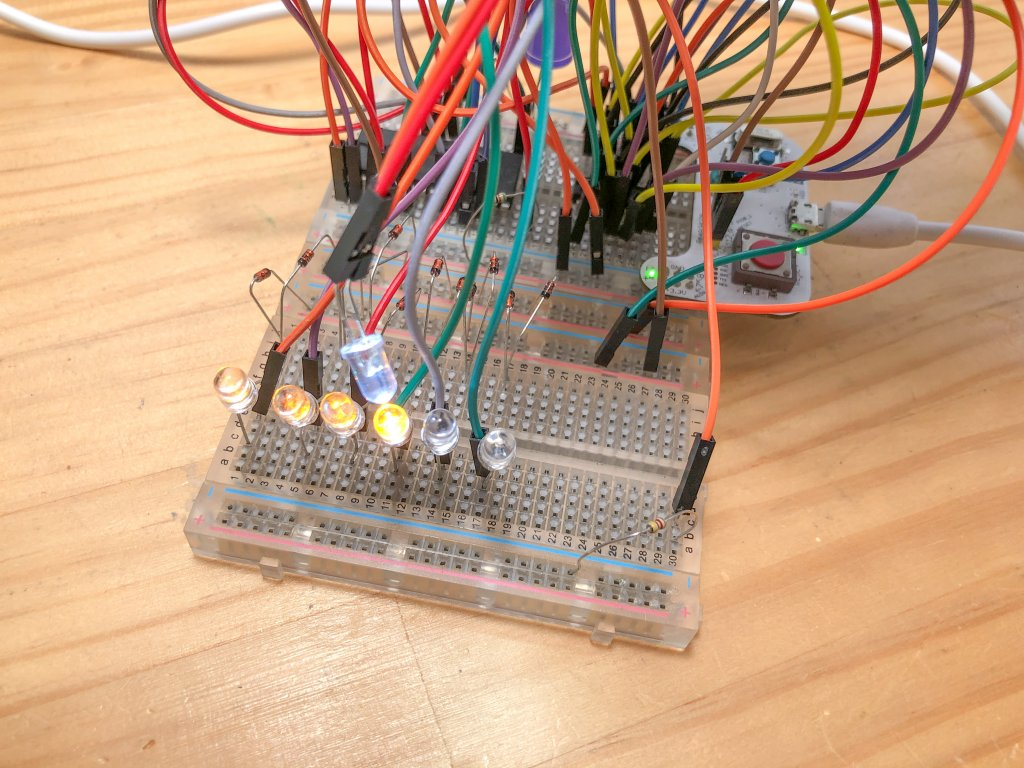The other day, I experimented with the use of LEDs as solar cells, taking advantage of the fact that the basic semiconductor structure is the same.

In this article, I would like to experiment with a "glowing light sensor" that can be used as both a light sensor and a glowing LED.

## Optical sensors using power generation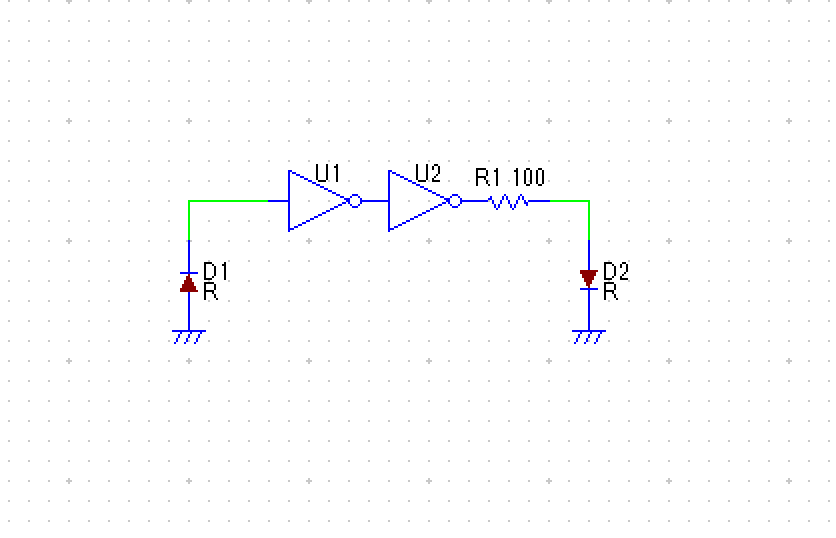I found out that when you shine light on an LED, it generates electricity, so I tried the circuit as a light sensor as shown above.

When light is applied to the LED on the left side of the schematic, the LED generates electricity and the voltage rises, and when the voltage exceeds the threshold voltage of the NOT gate, the NOT gate flips. There is another NOT gate, so I'll flip it further. So the resulting circuitry is that when the light hits the left side LED, the right side LED glows.

In this case, the light source is white LEDs and the light-receiving LEDs are yellow LEDs that are more responsive to white LEDs. I use 74HC04 for the NOT gate.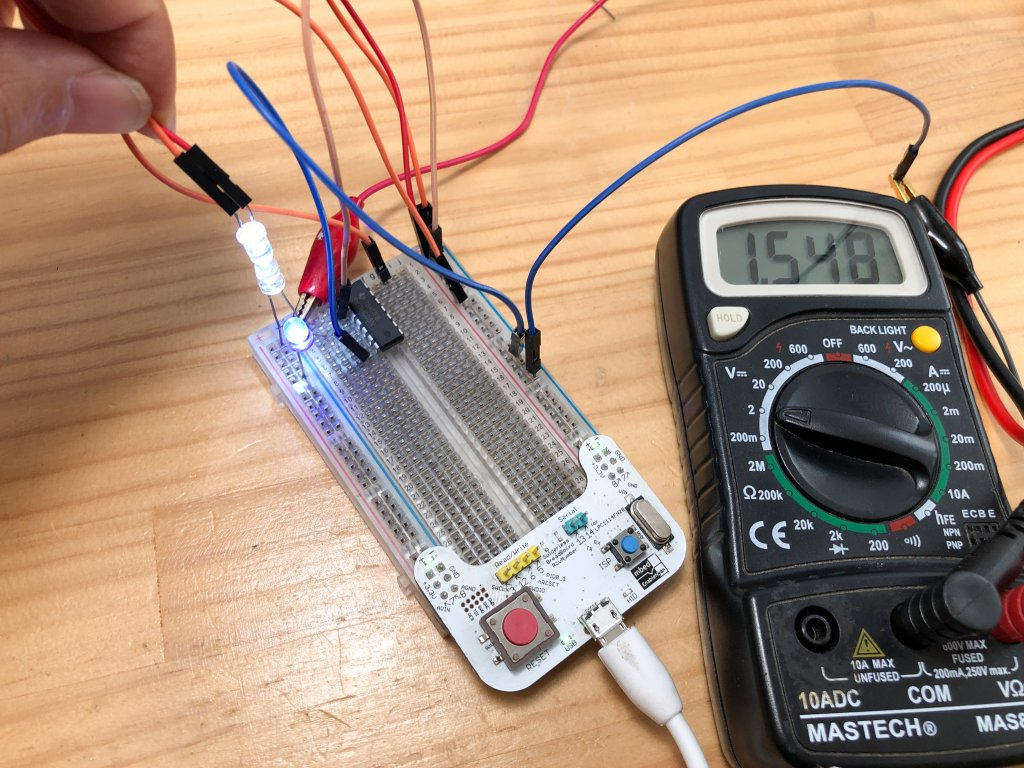As a result of the experiment, the voltage generated by the LEDs on the light receiving side is low, and the voltage does not easily exceed the threshold of the NOT gate.  The light source LED and the light receiving LED are exactly attached to each other and the reaction is finally achieved.

As a result, It is not very sensitive to be used as an optical sensor.

## Try to reverse bias the LED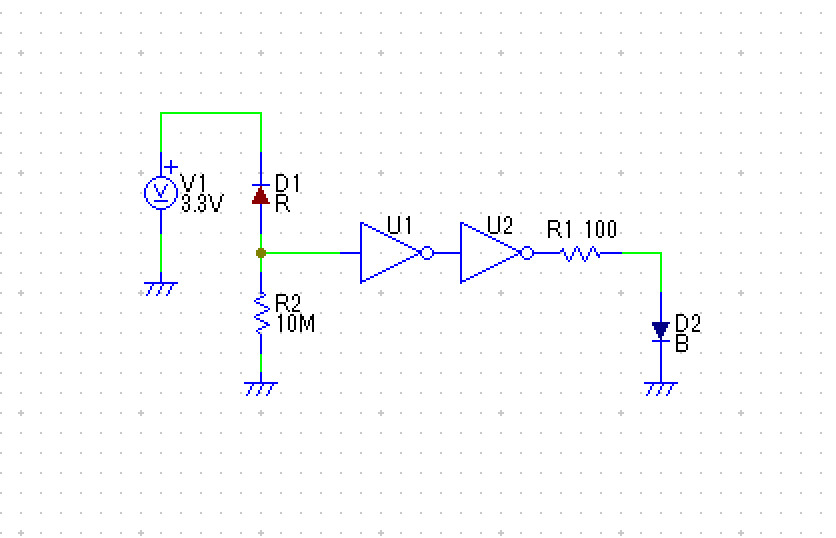This time, I'm going to experiment to see if it can be used as a sensor by setting the LEDs to reverse bias.  Reverse bias means that the negative pole (cathode) of the LED is connected to the power supply and the signal is obtained from the positive pole (anode), just like the LED on the left side of the circuit above.

Normally, the voltage at the negative pole (cathode) of the LED is high and the voltage at the positive pole (anode) is low, so current does not flow due to the characteristics of the diode and electricity does not flow to the anode.  Of course, the LED on the right side does not light up in this state.

In this circuit, if you shine light on the left LED on the light receiving side...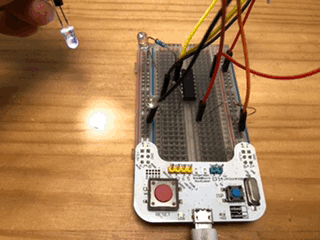The blue LED glowed. The LED on the light receiving side functions as an optical sensor.  The reverse bias circuit is more sensitive to light than the power generation circuit.

As the light hits the LED, its characteristics as a diode seem to weaken, causing some reverse bias current to flow out of it.

The input of the NOT gate was pulled down with a resistance of 10 MΩ.  It's a pretty high resistance, but it won't work consistently without it.  This is because, after reacting to the light, they remain responsive even when the light source is released. It seemed to accumulate electricity, so I needed a resistance to release that electricity.  However, if the resistance value is lower than 2MΩ, it will not work as an optical sensor.  Therefore, I set it to 10 MΩ.

## How to turn an LED into a "glowing light sensor"

By applying a reverse bias to the LED, it was found that it could be used as a light sensor.  Then I consider a circuit that can glow and can also be used as a light sensor.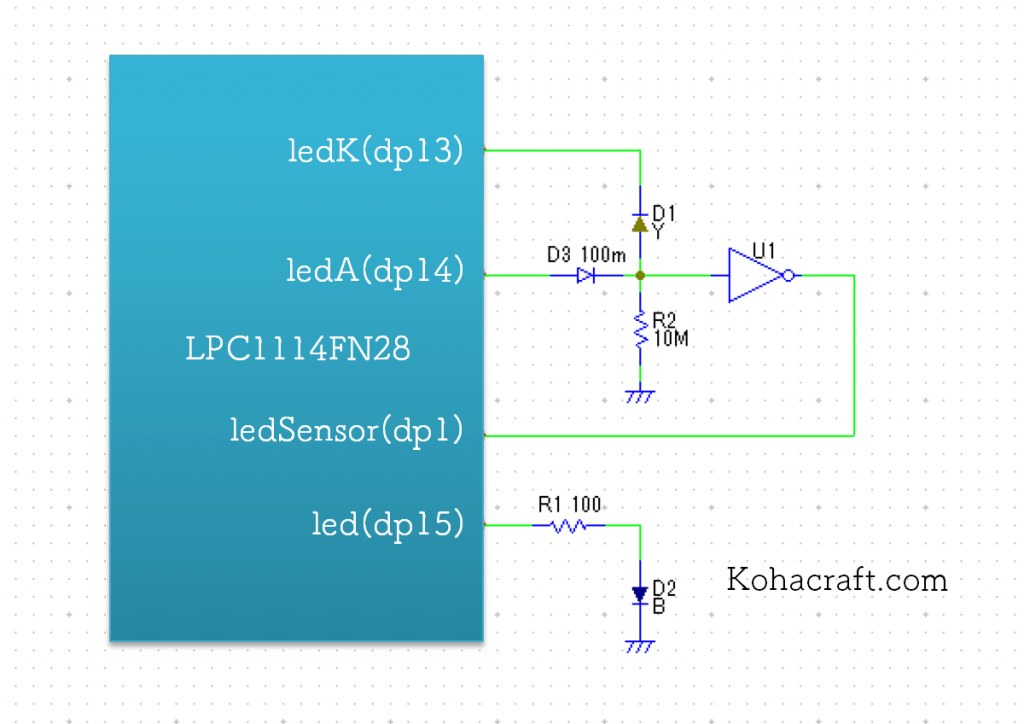The above is the circuit.  Because the logic IC is 3V, I used LPC1114, which operates at about 3V, instead of arduino for microcontroller.  The LPC1114 can be easily programmed using mbed.

We sell a convenient experimental set of LPC1114, a DIP-type microcontroller that is ideal for experimenting with a mbed by inserting and removing components from a breadboard. It's a great introduction to microcontrollers.### When using as an optical sensor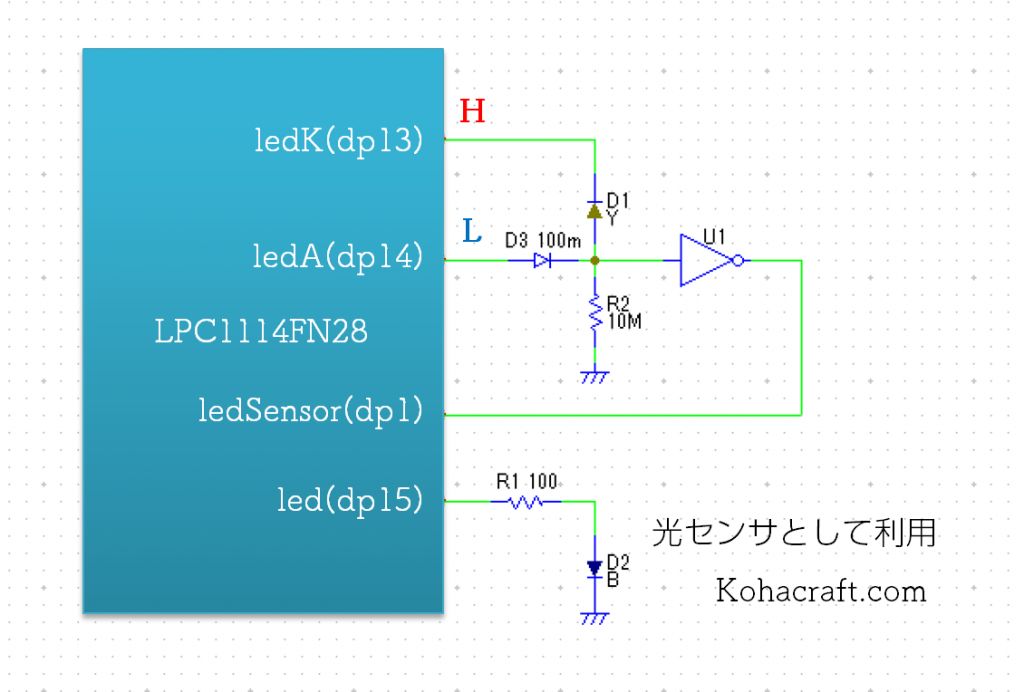When using it as an optical sensor, set the LEDK pin of the microcomputer to H and the LEDA pin to L.  In this way, the LED is set to a reverse bias state and the LED acts as a light sensor.

The result of the NOT gate is input to the microcontroller's ledSensor pin, and if it is L, it is judged to be responsive to light, and if it is H, it is judged not to be responsive to light.  (Since there is only one NOT gate, the results are reversed from the previous experiment.)

### When you shine the LED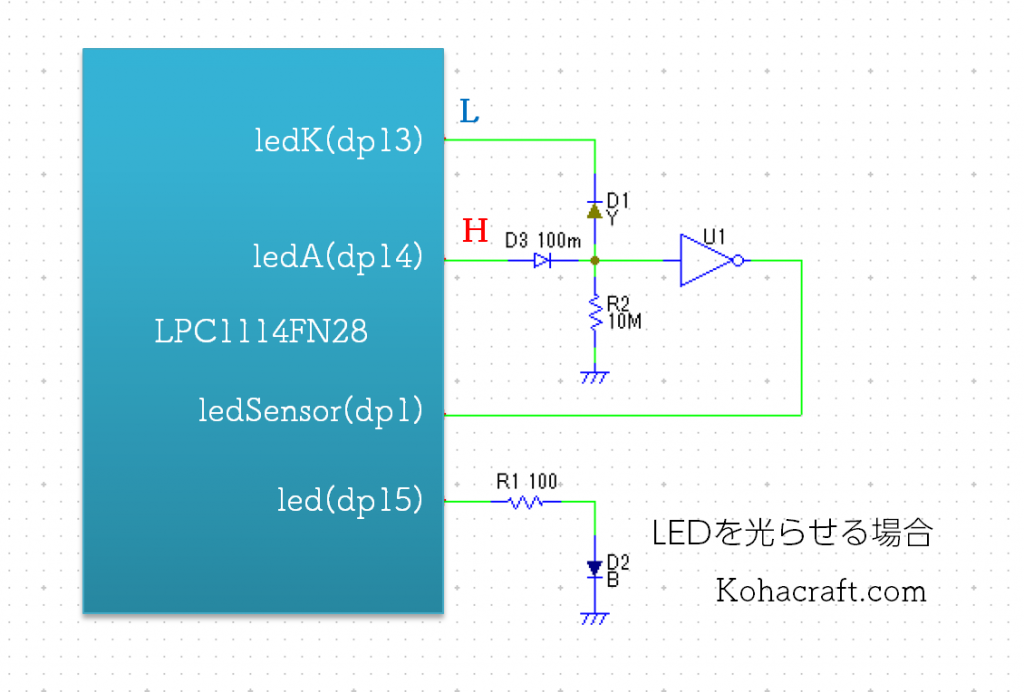If you want the LED to shine, set the pin of ledA to the "H" state. In this state, when the pin of the LEDK is set to "L", current flows through the LED and the LED lights up.  When the ledK pin is set to "H", no current will flow and the LED will turn off.

### Experiment with a program

Now that the mechanism has been decided, I will write a program.  Let the LED (yellow), which repeats on and off every 0.5 seconds, act as a light sensor for a moment every 0.1 seconds and output the measurement results to another LED (blue).

```#include "mbed.h"

DigitalOut ledK(dp13);
DigitalOut ledA(dp14);
DigitalIn ledSensor(dp1);

DigitalOut led(dp15);

bool lightDetect();

int main() {
while(1) {

for( int i=0 ; i<5 ; i ++ )
{
ledA = 1;   //Light sensor on
ledK = 0;
wait(0.1);
led = lightDetect();    //Output sensor status to LED
}

for( int i=0 ; i<5 ; i ++ )
{
ledA = 1;   //Light sensor off
ledK = 1;
wait(0.1);
led = lightDetect();    //Output sensor status to LED
}
}
}

//Sensing light as a light sensor
bool lightDetect();
{
ledA = 0;   //Set to light sensor mode
ledK = 1;
wait(0.001);    //1ms light sensor mode
bool sense = ledSensor; //Reading the status of a sensor

ledA = 1;   //set to light mode
return sense;
}
```

Here's the program.  The LED connected to dp13 and dp14 is an LED that is also used as a light sensor.  The LED connected to the dp15 is the output LED.

Let's see it in action.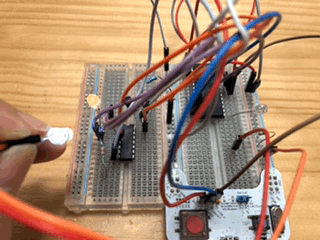The blinking yellow LED on the left is an LED that also serves as a light sensor.  When you move the white LED of the light source closer, the blue LED on the right side lights up in response.

LEDs can now be used as both a light sensor and a light-emitting device.  "Glowing light sensor" has been created.

By the way, when the NOT gate was omitted and the LED of the optical sensor was connected directly to the input pin of the LPC1114, it did not work as an optical sensor because the input impedance of the LPC1114 was low.

## Make many LEDs into a "glowing light sensor"

The finished "glowing light sensor" requires three ports per LED.  If you try to use a lot of "glowing light sensor" in your microcontroller, you will quickly run out of ports.

Therefore, I came up with a circuit to use as many "glowing light sensor" as possible with as few ports as possible.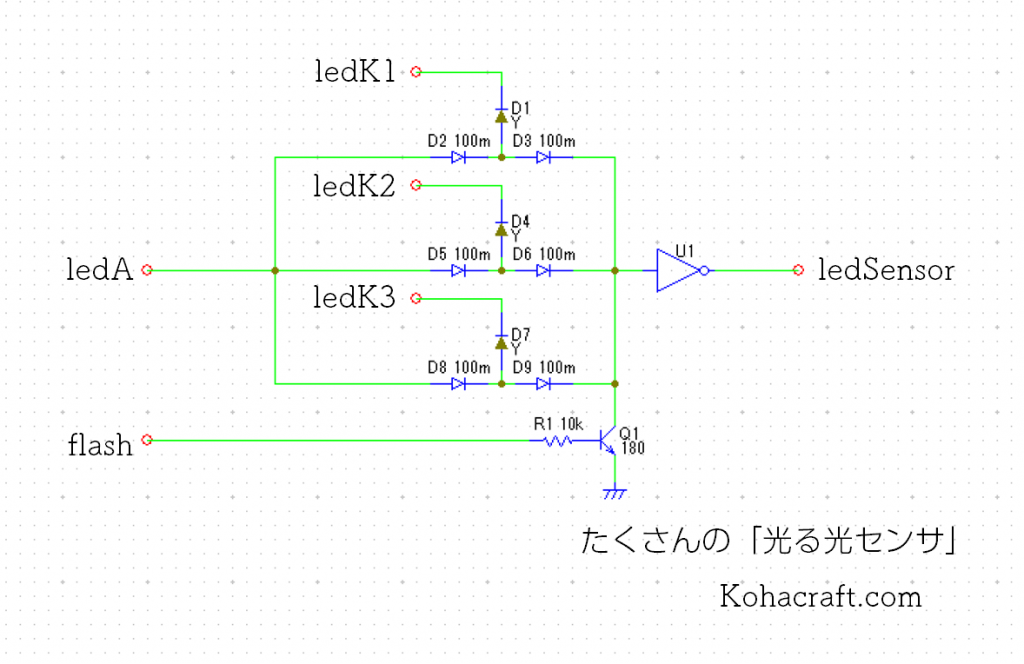### When using as an optical sensor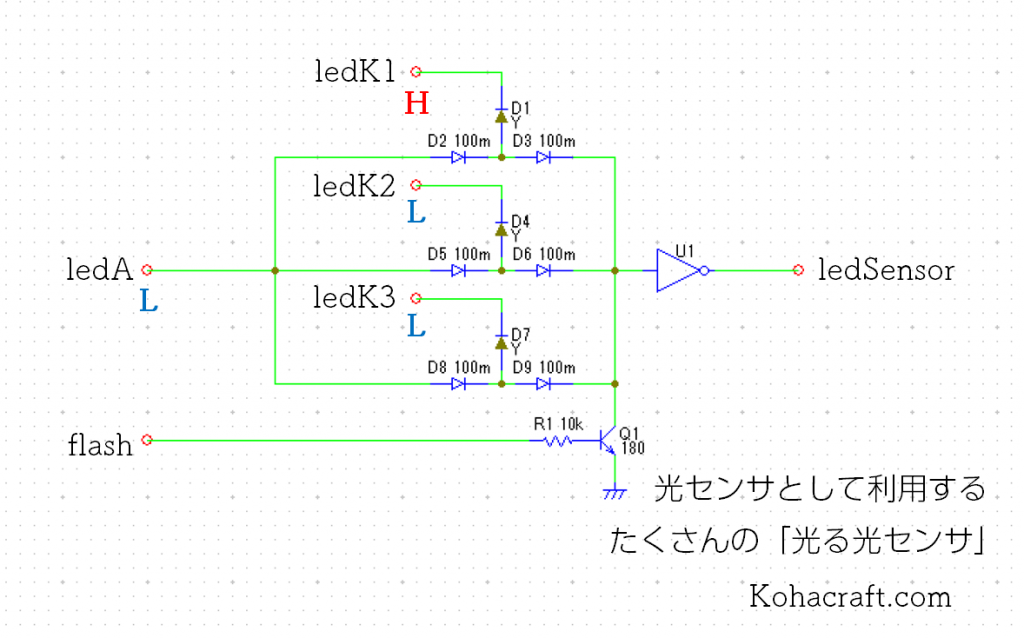To use the LEDA as a light sensor, set the pin of the LEDA to "L" as before.  Then set only the pin of the LED ledK you want to examine to H.  Now only certain LEDs will be reverse biased and become light sensors.

For example, when only ledK1 is set to H as shown in the above diagram, the LED of D1 functions as a light sensor, so the output of the Led Sensor is read to determine whether the LED of D1 is responsive to light.

Next, set only the ledK2 to H and read the output of the Led Sensor.  Next, I'm going to set only ledK3 to H... The scan is repeated for the number of LEDs in this way.

In the diagram above, the resistors that used to be 10MΩ have been replaced with transistors, and there is a new pin called "flash" that controls the transistors.  When I changed to the scanning method, the 10MΩ resistance did not discharge electricity in time when it reacted to light, and when one LED reacted, it was as if the other LEDs also reacted because the electricity was slow to discharge when one LED reacted.  Therefore, I made a circuit to force discharge.

Specifically,

• ledK1 H
• Read the result of the Led Sensor
• ledK1 L
• Flash for a moment H

In this way, I will scan all the LEDs ledK1 to ledKn.

### When shinning the LED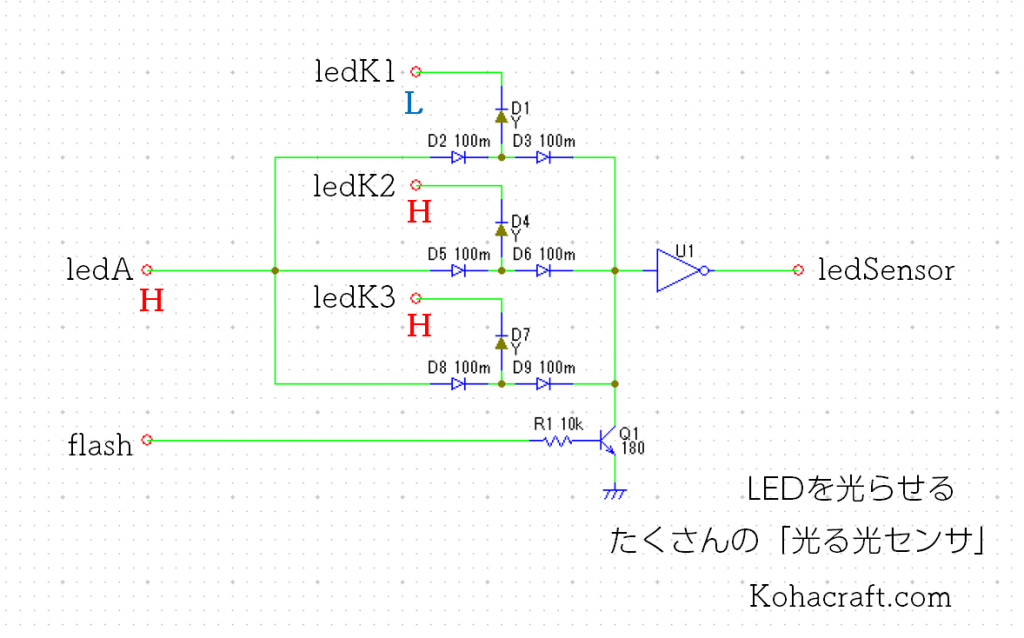If you want the LED to shine, set ledA to H.  Then, set only the LEDs you want to light up to "L" and set the LEDs you don't want to light up to "H".  By doing this, the current flows to the LED set to "L" and the LED lights up.  In the above example, only ledK1 is "L", so only the LED of D1 will light.

### I actually tried it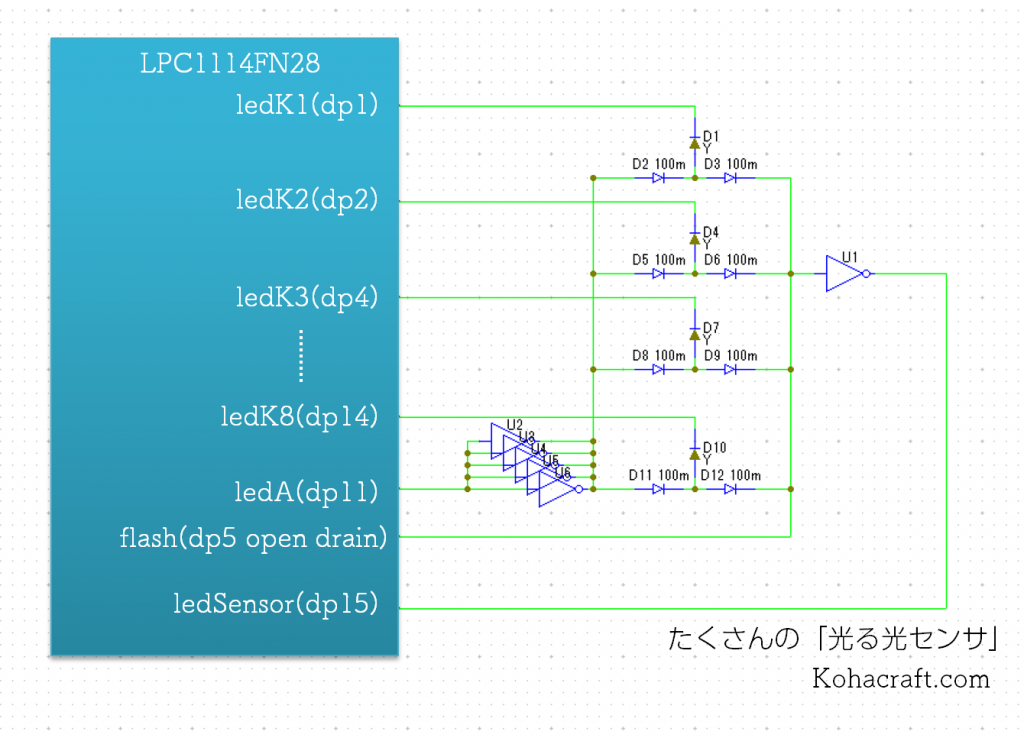I made it into 8 glowing light sensors.  This can be achieved with eight ledK1 to ledK8 and ledA, ledSensor and flash, for a total of 11 ports.

The program was as follows.

```#include "mbed.h"

DigitalOut ledK = { dp1, dp2, dp4, dp6, dp9, dp10, dp13, dp14};
DigitalOut ledA(dp11);

DigitalIn ledSensor(dp15);

bool sensorArray = { 0, 0, 0, 0, 0, 0, 0, 0 };
bool ledArray = { 0, 0, 0, 0, 0, 0, 0, 0 };

DigitalOut led(dp17);
DigitalOut flash(dp5);

void lightDetect();

int main() {

for( int i=0 ; i<8 ; i++ )
ledK[i] = 1;
flash = 1;

while(1) {

lightDetect();    //Get the sensor status

ledA = 0;   //Light sensor on
for( int i=0 ; i< 8 ; i++ )
{
if( sensorArray[i] == 1 )
ledArray[i] = 1;

if( ledArray[i] == 0 )
ledK[i] = 1;
else
ledK[i] = 0;
}
wait(0.01);
}
}

//Sensing light as a light sensor
void lightDetect();
{
ledA = 1;   //Set to light sensor mode
for( int j=0 ; j<8 ; j++ )  //Turn off all sensors
{
ledK[j] = 0;
sensorArray[j] = 0;
}
for( int i=0 ; i< 8 ; i++ )
{
flash = 0;
wait(0.0001);
flash = 1;

ledK[i] = 1;    //Turn on only certain sensors
wait(0.0001);
bool res = ledSensor; //Read the status of the sensor
if( res == 0 )
sensorArray[i] = 1;
else
sensorArray[i] = 0;
if( i== 3 )
led = sensorArray[i];
//wait(3);    //1ms light sensor mode
ledK[i] = 0;    //Turn off a specific sensor
}
return;
}
```

This is what happens when you actually make it work.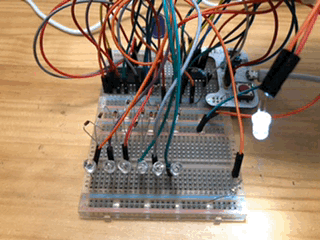You can see how the "glowing light sensor" lights up in response to the light source.

I've made a "glowing light sensor" using LEDs, so I'd like to play with it a bit.